Learn the 4 Things You Must Know Before Taking the GMAT!   Download it Now!

# GMAT Probability Questions

## Easier Strategy for Solving “Matrix” Problems

There is a special subset of GMAT probability problems often categorized as “matrix problems,” and they are particularly difficult for many students for a couple of reasons. First, students often don’t immediately recognize them for the type of question they are, often confusing them for “sets” problems or another type of GMAT word problem. Second, there are a lot of moving parts in these types of questions, and the standard solution method taught by many textbooks and prep courses — which is to make a matrix table and fill in the parts of the table accordingly — is often confusing and cumbersome.

Fortunately, there is a better way!

In this article, I’d like to teach what my students find to be an easier way to tackle these types of problems, which is to use what I call a “Probability Decision Tree”. For illustration, let’s look at the following example, adapted from one of my favorite GMAT textbooks, Chili Hot GMAT by Brandon Royal:

Question: In a college math course, 70 percent of the students are male and 30 percent of the students are married. If two-sevenths of the male students are married, what fraction of the female students is single?

(A) 2/7
(B) 1/3
(C) 3/7
(D) 2/3
(E) 5/7

In most questions of this type on the GMAT, there will be four combinations of possible outcomes. In other words, there will be a decision point with two possible outcomes, and then once you’ve picked one of those outcomes, there will be a second decision point with two additional possible outcomes. This complexity is best dealt with using a Probability Decision Tree, which enables you to visually draw out exactly what is happening and quantify each permutation accordingly. Here’s what I mean.

The first step when you see a problem like this is to isolate the two different decision points. In this hypothetical college math class, students are either:

• Male or Female
• Married or Single

Now, how should you start to account for the permutations of those different possible outcomes? Simply pick one of them and start drawing out the “branches” of your tree, as in Figure 1 on the right.

The question now becomes, how many of the students are Male and how many are Female? Well, the problem tells you that 70% of the students are male, which means that 30% are female. I like to illustrate that by writing those numbers, in decimal form (for easy multiplication, if necessary), on the branches of the tree, as follows: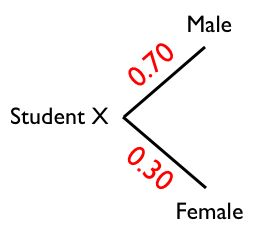Of course, as we’ve noted numerous times in various of our GMAT video lessons, it’s always best whenever possible to work with concrete numbers rather than in the abstract world of percentages and fractions. Therefore, it is best to assume that there are 100 total students in the class (when you’re dealing with percents, 100 is the best number to use because it makes the math so much easier). If we assume 100 students, then we can quantify exactly how many male (70) and female (30) students are in the class, as follows: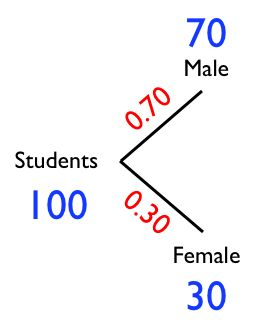Now, we come to our second decision point and can draw the remaining branches on our probability tree. Whether a student is male or female, he/she is either married or single. There are four such permutations, illustrated this way: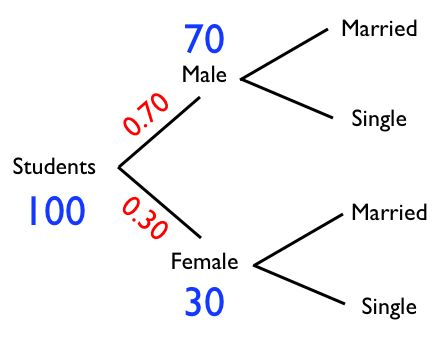The second sentence in the question tells us that 2/7 of the male students are married. The beauty of a probability decision tree like we’re using here is that it makes it super easy to make sure you’re separating out the students correctly. In this case we’re in the world of “male students,” so you start off on the upper branch of the tree. Then, we’re talking about married male students, so you denote that 2/7 of them are married as follows (you can convert 2/7 to 0.2857 if you want, but you’ll see that it’s actually easier to just leave it as a fraction at this point):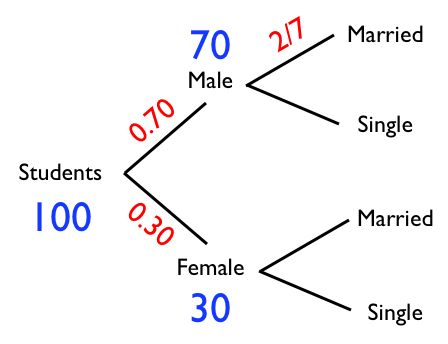Once again, it’s time to do a little math to quantify exactly how many male students are married. Since we’ve already determined, based on our hypothetical 100 total students, that 70 are male, then 2/7 of those 70 male students is 20. So, you’d want to write “20” in the appropriate place on your probability tree: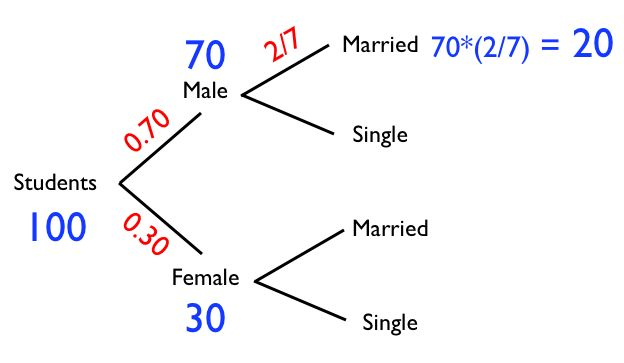The final piece of the puzzle tells us that 30 percent of the students are married. Since we’re assuming 100 total students, that means that 30 total students are married, right? But we’ve already accounted for some of those married students, haven’t we? The far right branches of our probability tree show two possible places for “married” students — Male–>Married and Female–>Married. We’ve already determined that there are 20 Male–>Married students, so that leaves how many Female–>Married students, if there are 30 total married students? Ten. Now you can fill in that part of the tree: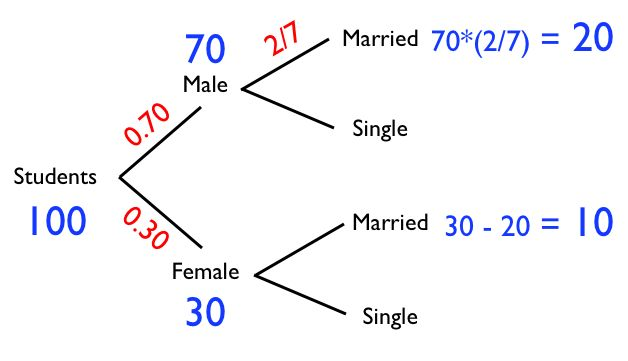The good thing about having converted esoteric percentages and fractions into tangible numbers is that it’s now easy to fill out the rest of the tree. If there are 70 total male students and 20 are accounted for, that means that 50 of them must be single. Likewise, if there are 30 total female students and 10 are accounted for, that means that 20 of the females are single: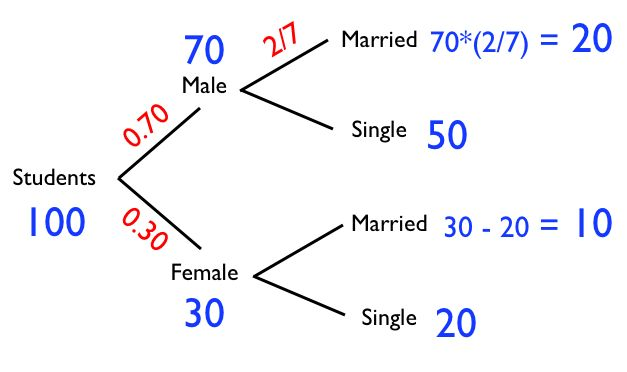That’s it! Now that we’ve broken all 100 students out into their respective categories, we can answer any questions that the GMAT might throw at us. In this case the question is, “What fraction of the female students is single?” Simply consult your Probability Tree and you’ll see that 20 of the 30 female students are single, so 20/30 reduces to 2/3, or Answer D. Great job if you got that!

Now, pop quiz: In this same question, what percentage of the class is married males?